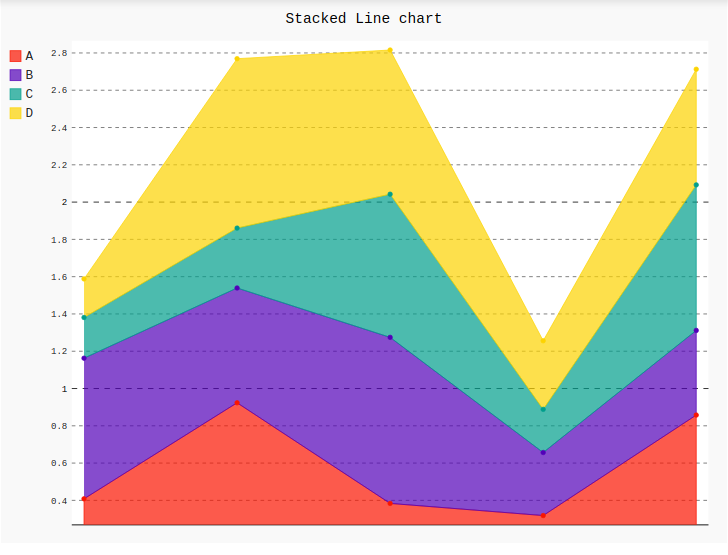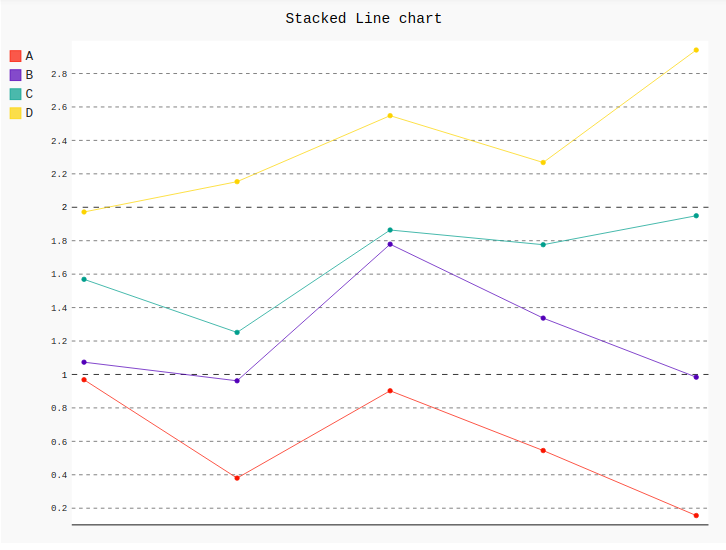GeeksforGeeks App
Open AppBrowser
Continue

# Stacked Line chart in Pygal

Pygal is a Python module that is mainly used to build SVG (Scalar Vector Graphics) graphs and charts. SVG is a vector-based graphics in the XML format that can be edited in any editor. Pygal can create graphs with minimal lines of code that can be easy to understand and write.

## Stacked line chart

A stacked line chart is a line chart in which lines never overlap because they amplify to each other points. Values are represented on the y-axis and categories are displayed on the x-axis. But the stacked line chart cannot present positive and negative value at the same time. Rendering helps to represent the graph more effectively.

Syntax:

`line_chart = pygal.StackedLine(fill=True)`

Example 1:

 `# importing pygal``import` `pygal``import` `numpy`` ` ` ` `# creating line chart object``line_chart ``=` `pygal.StackedLine(fill ``=``"True"``)`` ` `# naming the title``line_chart.title ``=` `'Stacked Line chart'`` ` ` ` `# adding lines``line_chart.add(``'A'``, numpy.random.rand(``5``))``line_chart.add(``'B'``, numpy.random.rand(``5``))``line_chart.add(``'C'``, numpy.random.rand(``5``))``line_chart.add(``'D'``, numpy.random.rand(``5``))`` ` `line_chart`

Output:Example 2:

 `# importing pygal``import` `pygal``import` `numpy`` ` ` ` `# creating line chart object``line_chart ``=` `pygal.StackedLine()`` ` `# naming the title``line_chart.title ``=` `'Stacked Line chart'`` ` ` ` `# adding lines``line_chart.add(``'A'``, numpy.random.rand(``5``))``line_chart.add(``'B'``, numpy.random.rand(``5``))``line_chart.add(``'C'``, numpy.random.rand(``5``))``line_chart.add(``'D'``, numpy.random.rand(``5``))`` ` `line_chart`

Output:My Personal Notes arrow_drop_up